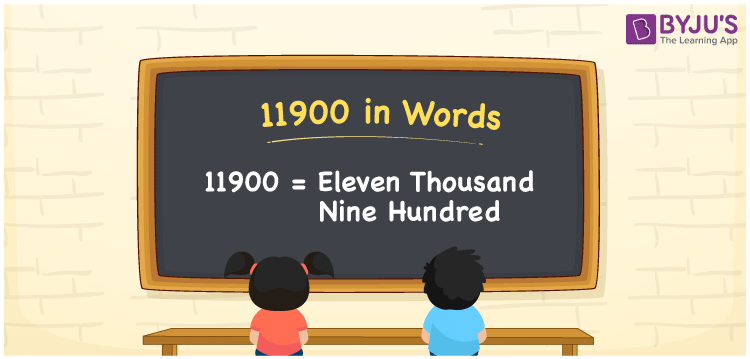# 11900 in Words

The number 11900 in words is written as Eleven Thousand Nine Hundred. Generally, ones, tens, hundreds, thousands places are used to write the number names. Hence, a place value chart is important to write the number 11900 in words. For instance, if you saved Rs. 11900 in three months, you can write, “I have saved Rupees Eleven Thousand Nine Hundred in three months”. 11900 is a cardinal number. In this article, let us learn how to write and spell the number name of 11900.

 11900 in words Eleven Thousand Nine Hundred Eleven Thousand Nine Hundred in numerical form 11900

## 11900 in English Words

Generally, numbers in words are written using the English alphabet. Hence, 11900 in words is written as Eleven Thousand Nine Hundred.## How to Write 11900 in Words?

Determine the place value of each digit to write the number 11900 in Words. The place value chart for the number 11900 is shown below:

 Ten-Thousands Thousands Hundreds Tens Ones 1 1 9 0 0

Hence, the extended form of 11900 is written as:

1 x Ten Thousand + 1 x Thousand + 9 x Hundred + 0 × Ten + 0 × One

= 1 x 10000 + 1 x 1000 + 9 x 100 + 0 + 0

= 10000 + 1000 + 900

= 11900

= Eleven Thousand Nine Hundred

Therefore, 11900 in words is written as Eleven Thousand Nine Hundred.

Interesting way of writing 11900 in words

1 = One

11 = Eleven

119 = One Hundred and Nineteen

1190 = One Thousand One Hundred Ninety

11900 = Eleven Thousand Nine Hundred

Thus, the word form of the number 11900 is Eleven Thousand Nine Hundred.

11900 is a natural number. The successor of 11900 is 11901 and the predecessor is 11899

• 11900 in words – Eleven Thousand Nine Hundred
• Is 11900 an odd number? – No
• Is 11900 an even number? – Yes
• Is 11900 a perfect square number? – No
• Is 11900 a perfect cube number? – No
• Is 11900 a prime number? – No
• Is 11900 a composite number? – Yes

## Frequently Asked Questions on 11900 in Words

Q1

### How to write 11900 in words?

11900 in words is written as Eleven Thousand Nine Hundred.
Q2

### Simplify, 10000 + 1900, and express in words.

On simplifying 10000 + 1900, we get 11900. Hence, 11900 in words is Eleven Thousand Nine Hundred.
Q3

### Write Eleven Thousand Nine Hundred in numbers.

Eleven Thousand Nine Hundred in numbers is 11900.
Test your Knowledge on 11900 in Words